# DataWeave map function: How to iterate through all items in an Array

10 min read | Last updated on Dec 2022

We would like to thank MuleSoft Ambassador Joshua Erney for their contribution to this developer tutorial.

In this tutorial, you’ll learn some examples or use cases in which you can iterate through all the items in an Array to change them. If you come from a different development background, you may know this function as `for` or `forEach`. Since DataWeave is a functional programming language, the statements are not being executed in a sequence (like a `for` would). Follow this tutorial to understand how to use `map` to achieve the same output.

To learn more about the differences between DataWeave as a functional programming language and imperative programming, check out the documentation.

You can try all of these examples with the DataWeave Playground. To learn more about it, check out this tutorial.

## Prerequisites

While not required to follow this tutorial, a good understanding of the basic DataWeave concepts would be preferred. You can check out these other tutorials if you feel a bit lost with some concepts:

## Syntax

The `map` function satisfies a very common use case in integration development: transforming every item in an Array to something else. `map` takes two parameters: an Array and a lambda.

There are two type variables in this definition: `T` and `R`. `T` represents the type of items that the input Array contains. `R` represents the type of items that the output Array contains. Since `map`’s job is to transform every item in an Array, it makes sense that the type of items in the input Array and the type of items in the output Array can be different. Knowing this, the lambda definition makes sense:

The lambda’s job is to take in each item of type `T` from the input Array, as well as the index of that item, and return a new item that will be used in the output Array.

## Prefix Notation

This function is not commonly used with the prefix notation because of its complexity. But here you can find an example that adds `1` to every value in the input Array.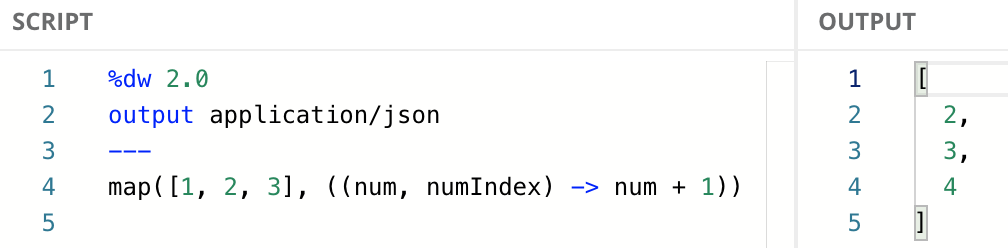## Infix Notation

This is the most used notation for this function because it makes it easier to read and understand.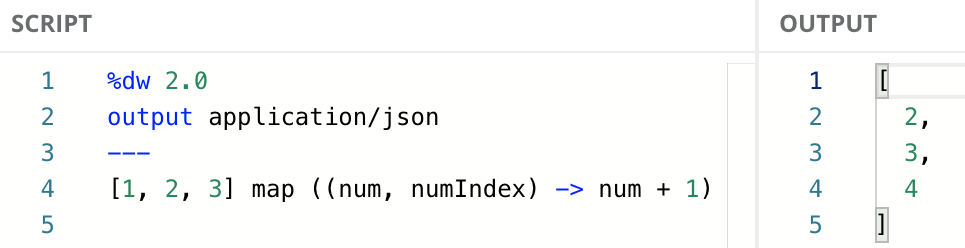When using the infix notation, you don’t have to include all the parameters in the lambda if you’re not using them. However, you do have to include the previous parameters (to the left) whether you’re using them or not. You can only remove the parameters to the right.

In this case, since `num` is the first parameter, we can also use the following code:

## Dollar-sign Syntax

You don’t always need to include the whole explicit lambda expression as the second argument. You can use the dollar-sign syntax to reference the two arguments that are passed to the lambda (i.e., `num` and `numIndex`). In this example, we are adding the value of each item plus the index of each item. `\$` represents the value of the item (`num`), while `\$\$` represents the index (`numIndex`).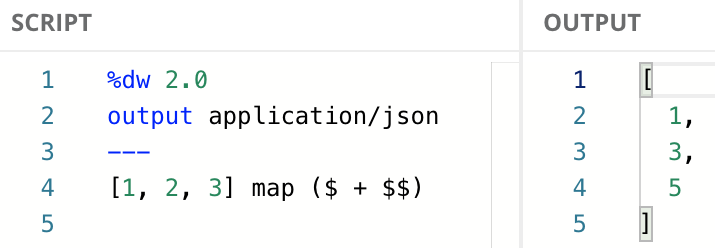The same example with the explicit lambda can be written like this:

## Modify the Items in an Array of Strings

Let’s check out a simple example of `map` in action. This script adds `"Hello, "` to the beginning of the String in the input Array.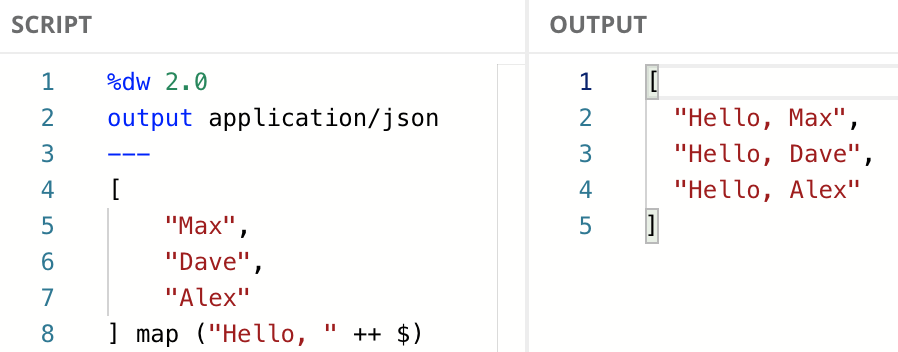If you’re not familiar with the `++` function, it can be used with two Strings to concatenate them into a new String. All the different ways to use `++` are out of scope for this tutorial, but you can learn more about it in the MuleSoft documentation or this developer tutorial.

In this example, we used the infix notation and the dollar-sign syntax. We chose to write it this way because the code looks cleaner. If you wanted to use explicit lambda, while still using the infix notation, the same code would look like this:

## Enrich Existing Data

One common use case is enriching existing datasets with more data. For example, if we have an input Array of Numbers that we want to transform into an Array of Objects with two fields: `number` and `type`. The `type` field would give us more information about the number: whether it’s an odd or even number.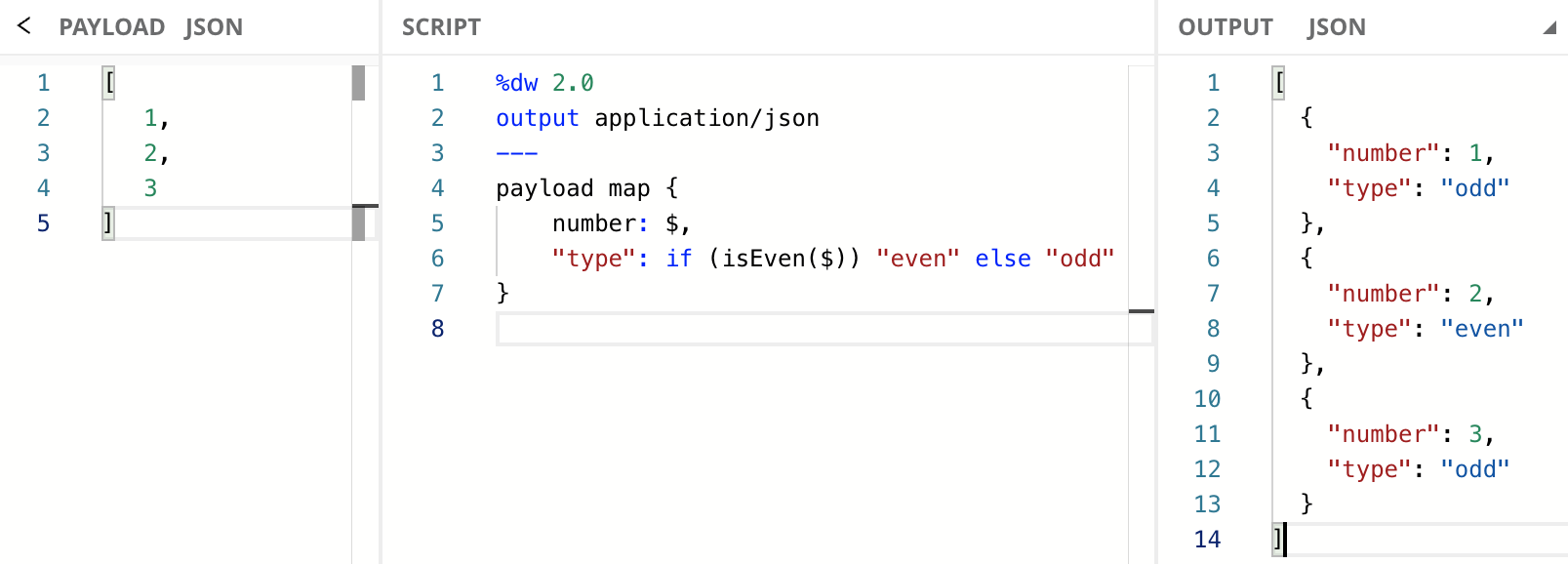To learn more about the `isEven` function, check out the documentation.

Notice how in the previous example we used `map` with parentheses (`map (...)`) but now we used curly brackets (`map {...}`). The difference is that in the first example we just wanted to modify the existing values into something different. However, in the second example, we wanted to create an Object. Objects in DataWeave are created using curly brackets and you need to provide a key/value structure (`map { key: value }`).

## Remove Unnecessary Data

Another common use case is paring down existing datasets to only contain the data that you’re interested in.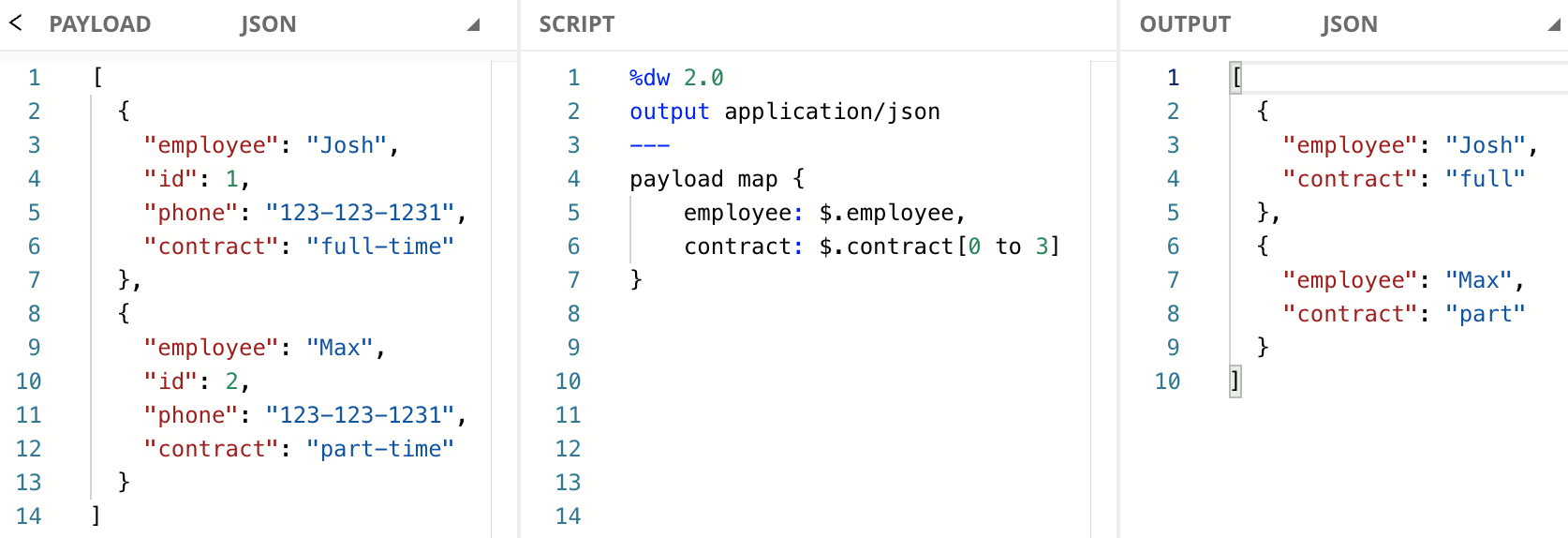To learn more about the Range Selector (`[0 to 3]`), check out the documentation or this developer tutorial.

## Use the Index as a Key

Some use cases need to use each item’s index. We already saw how to use the index in the value. Let’s now see some examples to use the index as a key for our output Object.

### Explicit lambda

First, let’s see how this solution would look like when using explicit lambdas.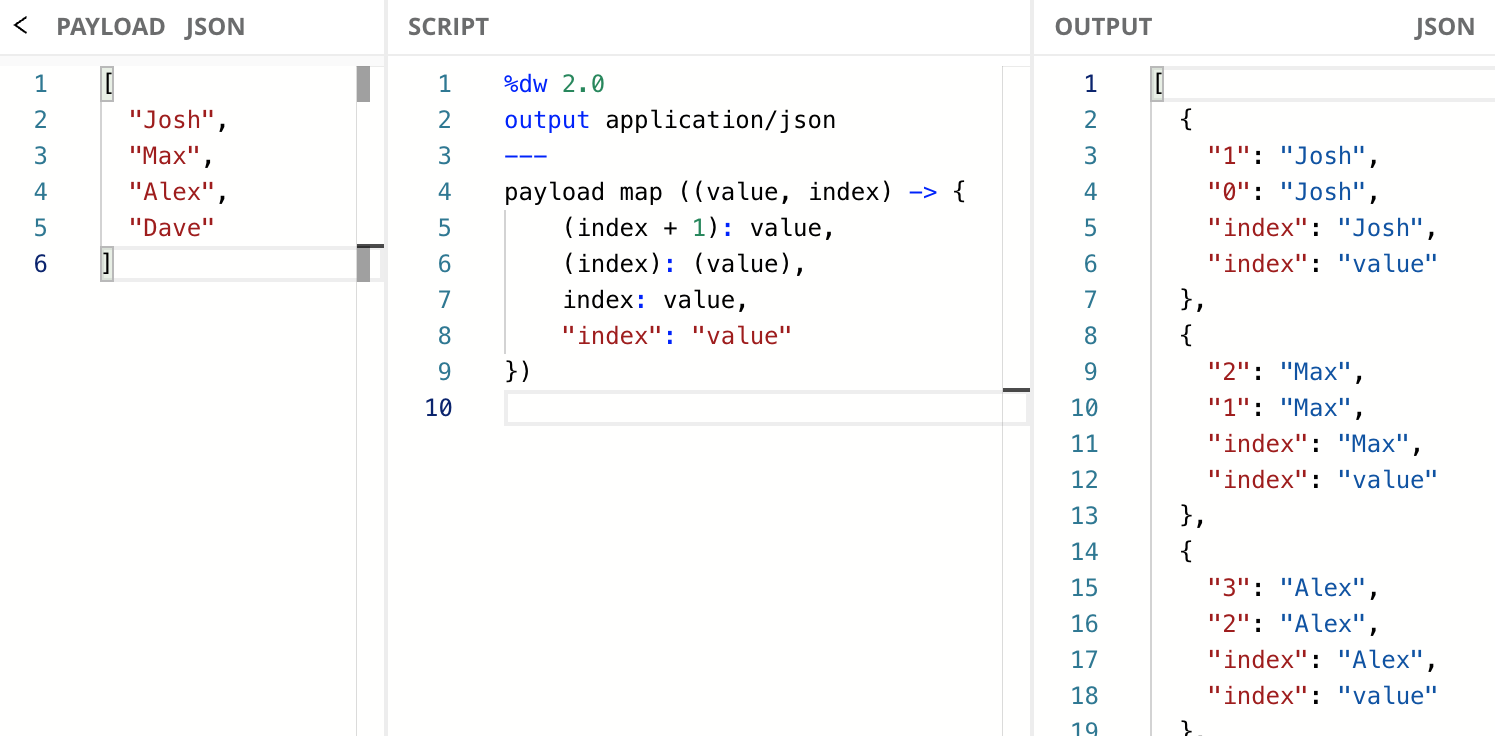We wanted to use the `index` to make a list of the names we had on the input. Since we wanted this output to start at `1` and not at `0`, we had to include a `+ 1` operation in the key portion of the Object.

You need to surround the `index` parameter in parentheses to correctly reference the value of the index. If you don’t do this, then the String `"index"` will be taken instead.

### Dollar-sign syntax

If your preference is to use the dollar-sign syntax, here is how you can use `\$\$` to reference the index from the key portion of the Object.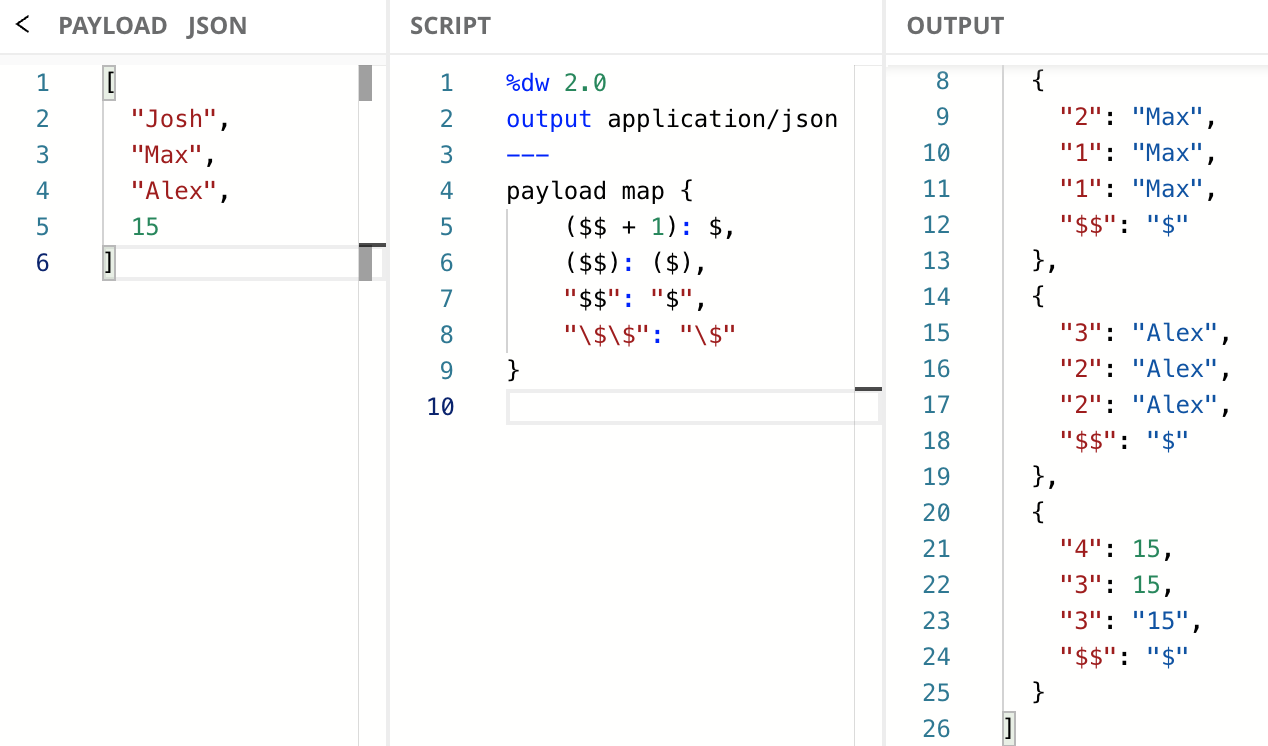You might be wondering why is the last item from the input Array a `15` instead of a String. This can give us a better picture of how the quotes will work on this syntax. If we use `"\$"` in the value portion of the Object, the Number `15` gets transformed into a String `"15"`. Note that using this syntax we can correctly reference the index just by using `"\$\$"`, whereas in the last example, `"index"` gave us the hardcoded value instead of the reference.

In this tutorial, you learned how to use the `map` function and some examples of how you can apply it. As opposed to other languages like Java or Python, DataWeave is a functional programming language that does not have a `for` or `forEach` function. Instead, you can achieve a similar output with `map` by iterating through all the values in the Array and modifying it accordingly.